Function Repository Resource:

# ConditionalCategoricalDistribution

Obtain a conditional categorical distribution formed by restricting the domain of a categorical distribution

Contributed by: Seth J. Chandler
 ResourceFunction["ConditionalCategoricalDistribution"][{pos1→pattern1,pos2→pattern2,…},dist] restricts the domain of dist to those elements whose value at each posi matches patterni. ResourceFunction["ConditionalCategoricalDistribution"][{pattern1,pattern2, …},dist] restricts the domain of dist to those elements whose value at position i matches patterni.

## Details and Options

Use _ as the pattern in order to let any value be acceptable at a given position of a domain element.
The pattern may be specified using a List of patterns whose length is shorter than the length of each domain element. When this happens, the function right-pads the List with _.
By default, if the result of the function is a univariate distribution, the resulting domain elements do not each have List wrapped around them. To wrap each domain element in List, set the "FlattenUnivariate" option to False.
By default, if a dimension of the result has only one possible value, the distribution "marginalizes out" that dimension. Setting the option "Marginalization" to False prevents that behavior.

## Examples

### Basic Examples (4)

 In:=Out=Condition that distribution on the first dimension taking on a value of "A":

 In:=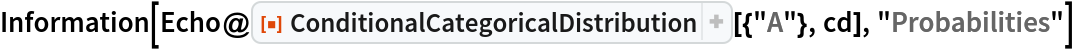Out=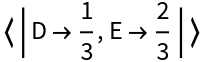Find the probabilities from that categorical distribution conditioned on the second dimension taking on a value of "E":

 In:=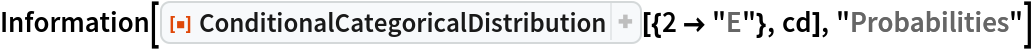Out=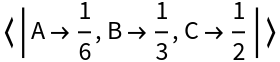Use a list of patterns instead of rules to impose the same condition as above:

 In:=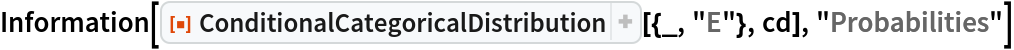Out=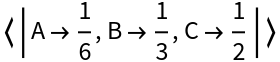### Scope (3)

Patterns used in the conditions can be complex:

 In:=Out=In:=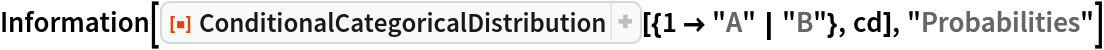Out=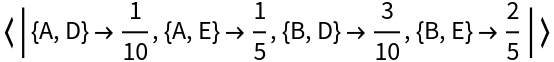The first category must be a letter in the word "ABLE":

 In:=Out=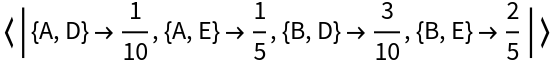A CategoricalDistribution of arbitrary dimension works with the function:

 In:=Out=In:=Out=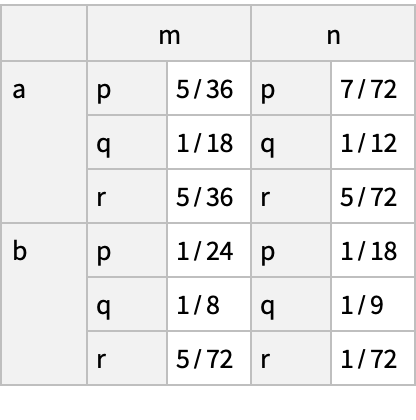The function works with univariate categorical distributions returning a CategoricalDistribution with potentially fewer categories:

 In:=Out=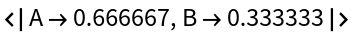### Options (2)

The value of the "FlattenUnivariate" option (True by default) determines whether the result of a univariate ConditionalCategoricalDistribution has its categories described without a List wrapper:

 In:=Out=In:=Out=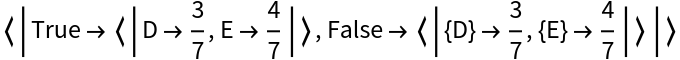Setting the "Marginalize" option to False preserves the dimensionality of the original distribution:

 In:=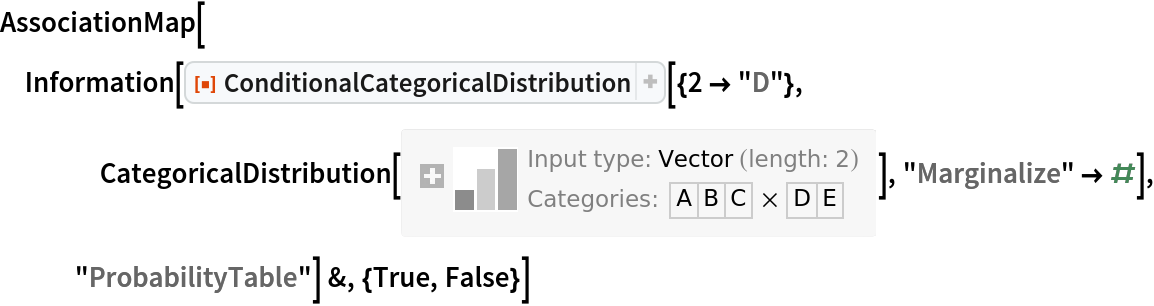Out=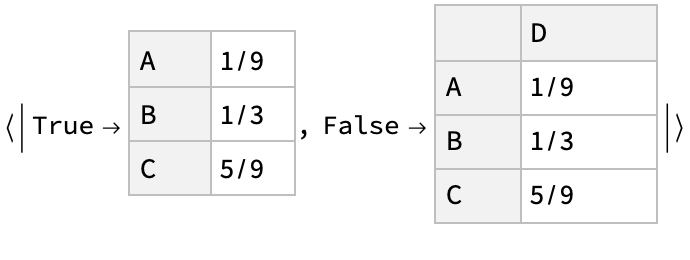### Applications (2)

Here is the joint distribution of persons in group A or B who have or do not have some disease, and to whom a test classifies as negative or positive for the disease. Find the joint distribution of persons in group A with respect to disease and test result:

 In:=Out=In:=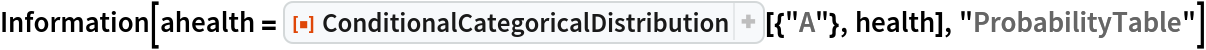Out=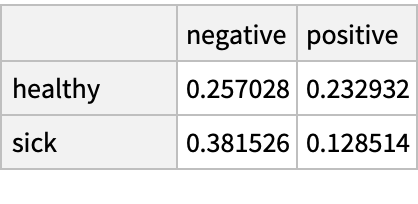Find the probability that a person in group A who tests positive is actually sick:

 In:=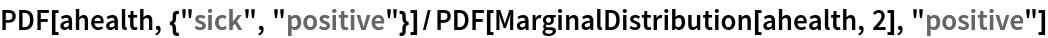Out=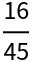Compute the fractions of true positives (sensitivity), true negatives (specificity) and false positives (1-specificity) for a mixture of categorical distributions:

 In:=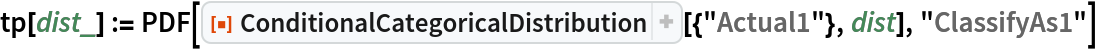In:=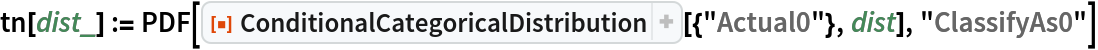In:=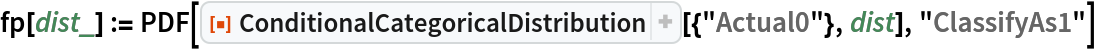In:=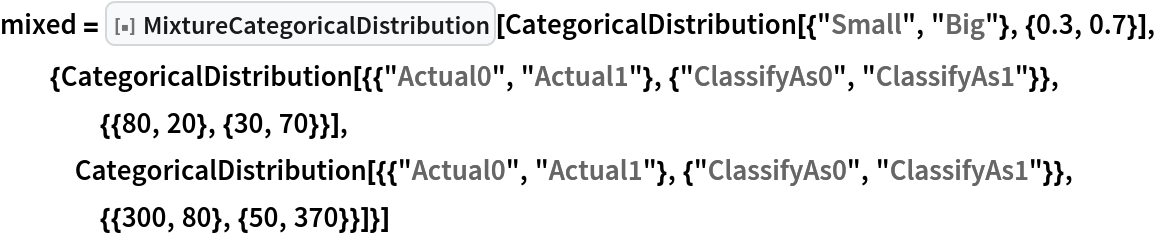Out=In:=Out=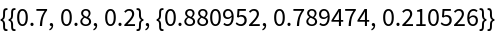### Properties and Relations (1)

The resource function MixtureCategoricalDistribution increases the dimensionality of a CategoricalDistribution, whereas ConditionalCategoricalDistribution reduces the dimensionality:

 In:=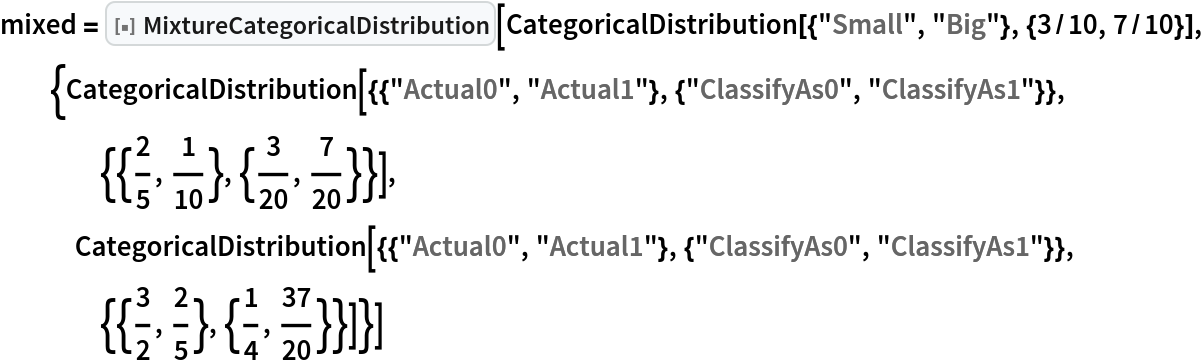Out=In:=Out=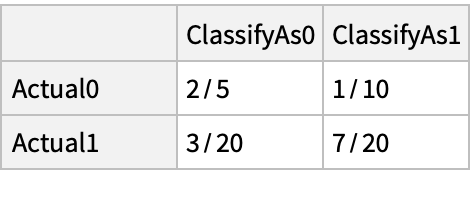### Neat Examples (4)

The following application comes from the field of causal inference, which is sometimes referred to as "do-calculus". Assume the joint probability distribution of the size of a kidney stone, the treatment one receives for it and how the outcome of that treatment is distributed as set forth below:

 In:=Compute the probability of a good outcome conditioned on the treatment. It will appear that B has better outcomes than A:

 In:=Out=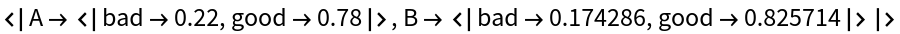Now synthesize a randomized controlled trial and derive the interventional distribution when one forces the treatment to be "A" by computing a MixtureCategoricalDistribution over stone size in which the components are the conditional categorical distributions based on the stone size and the treatment being A:

 In:=Out=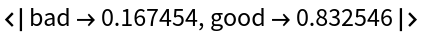Do exactly the same thing but force the treatment to be "B":

 In:=Out=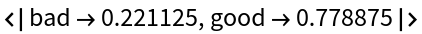Although the observational distribution might suggest treatment B is superior, in fact, treatment A is superior and the observational distribution is distorted by the fact that small kidney stones, which generally have better outcomes, are more frequently treated with treatment B.

Seth J. Chandler

## Version History

• 2.0.0 – 02 August 2021
• 1.0.0 – 21 May 2020